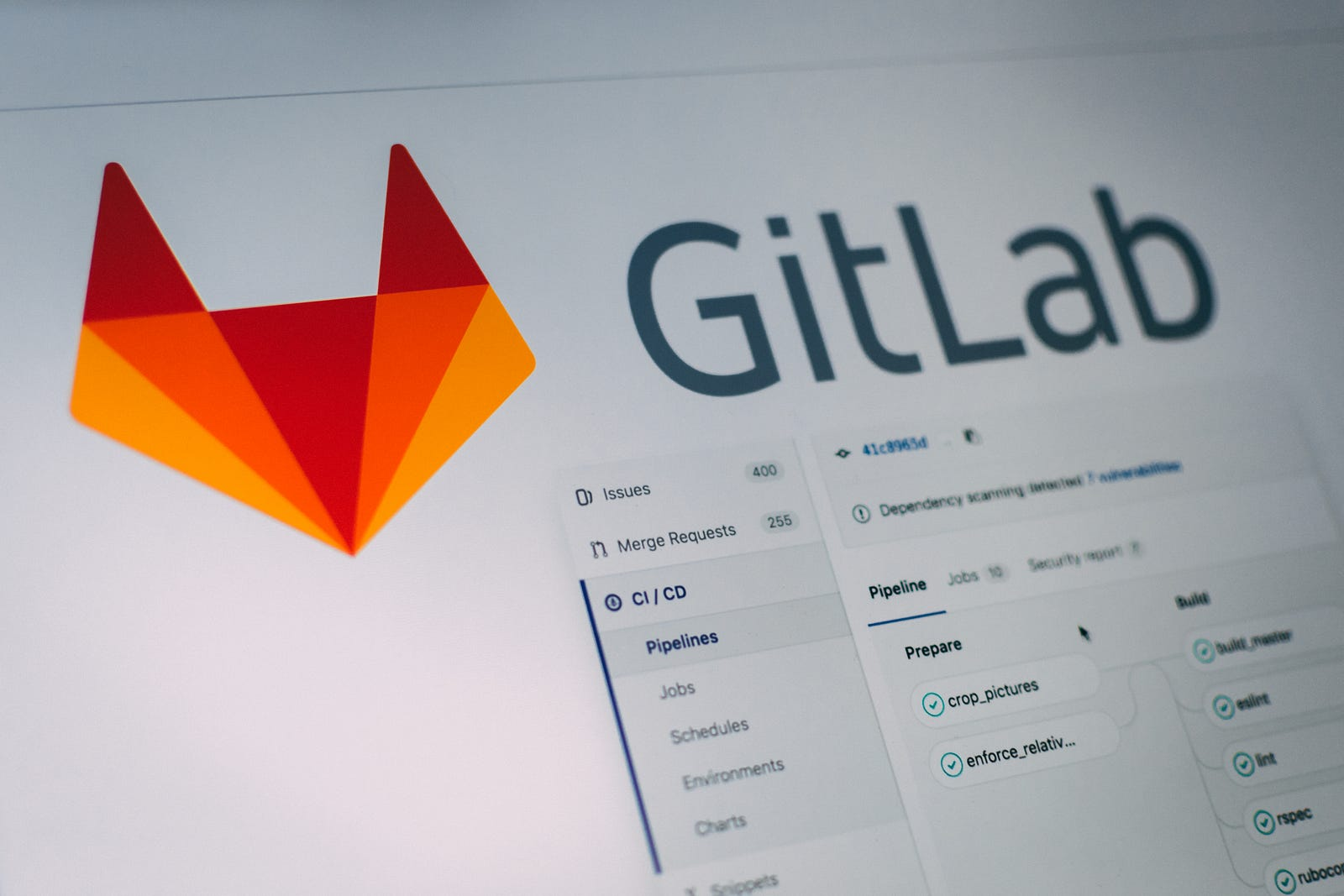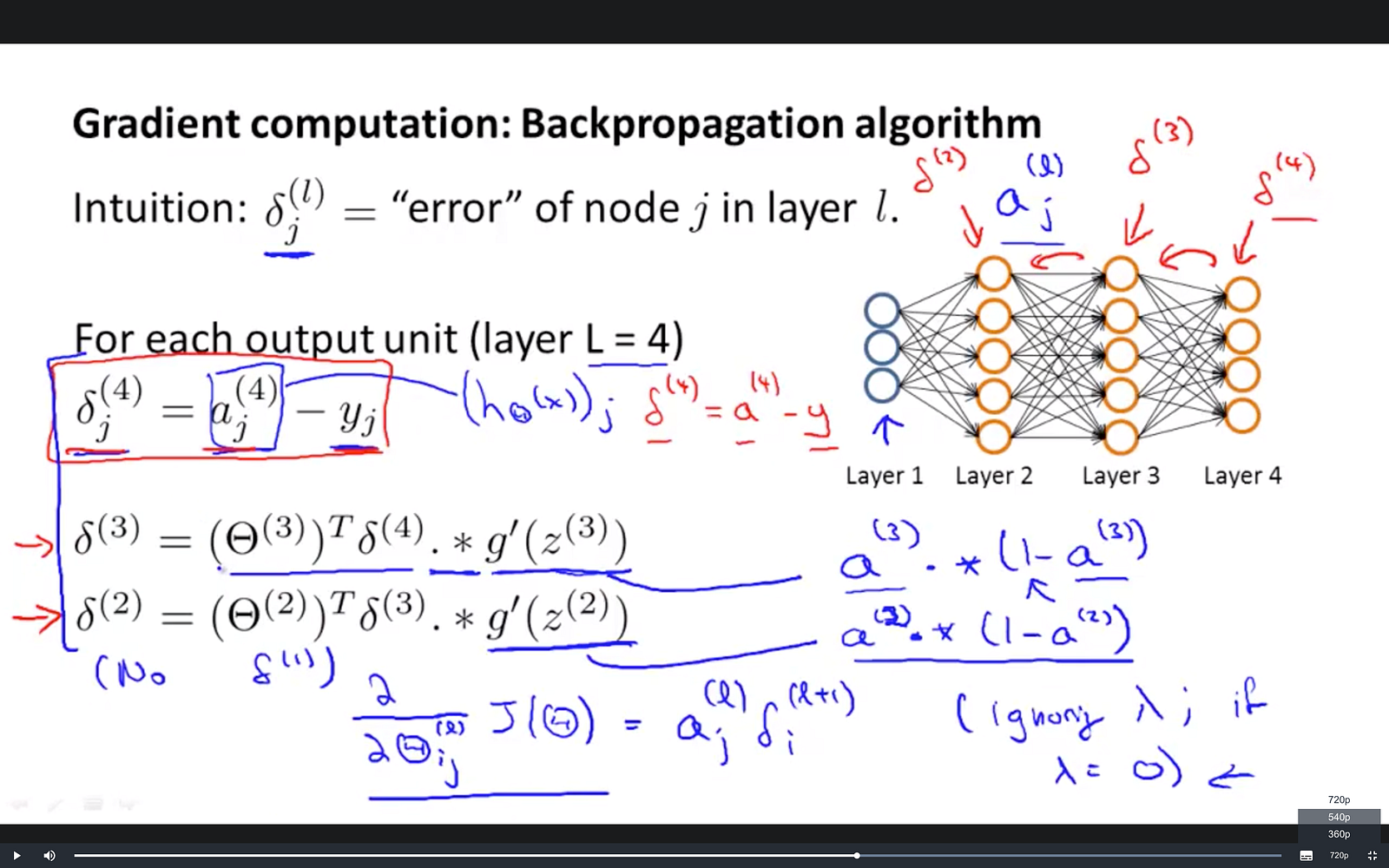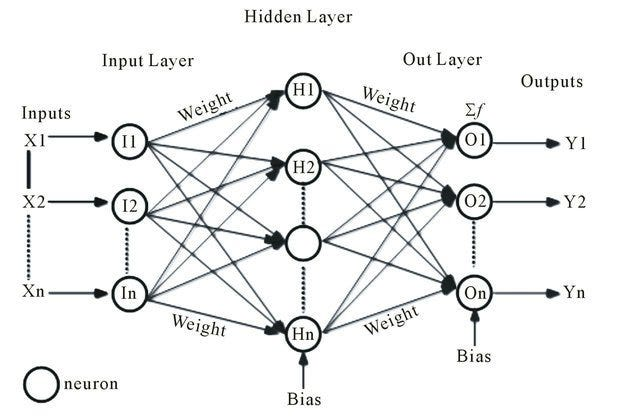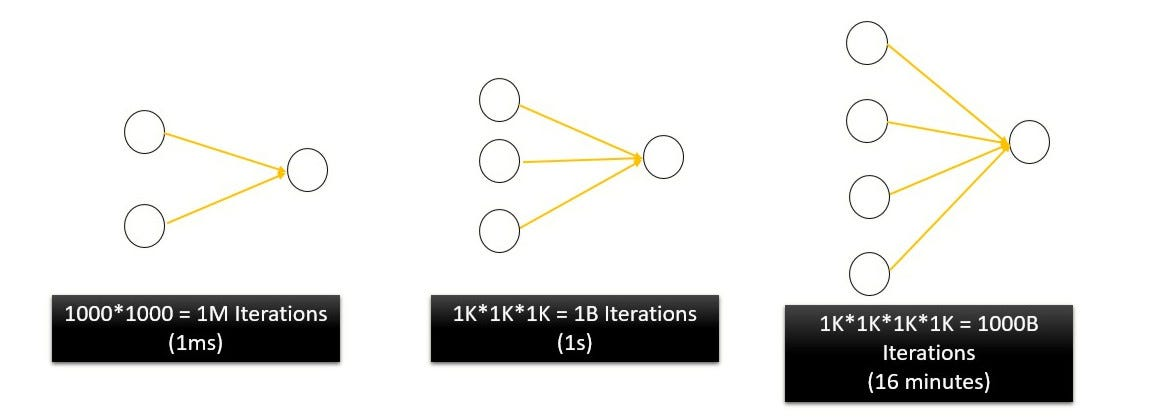# Layman’s Introduction to Backpropagation

## Training a neural network is no easy feat but it can be simple to understand it

Backpropagation is the process of tuning a neural network’s weights to better the prediction accuracy. There are two directions in which information flows in a neural network.

1. Forward propagation — also called inference — is when data goes into the neural network and out pops a prediction.
2. Backpropagation — the process of adjusting the weights by looking at the difference between prediction and the actual result.Photo by Pankaj Patel on Unsplash

Backpropagation is done before a neural network is ready to be deployed in the field. One uses the training data, which already has known results, to perform backpropagation. Once we are confident that the network is sufficiently trained we start the inference process.

These days backpropagation is a matter of using a single command from a myriad of tools. Since, these tools readily train a neural net most people tend to skip understanding the intuition behind backpropagation. Understandably so, when the mathematics looks like this.Andrew Ng’s Coursera course: https://www.coursera.org/learn/machine-learning

But it makes a lot of sense to get an intuition behind the process that is at the core of so much machine intelligence.

#### The role weights play in a neural network

Before trying to understand backpropagation let’s see how weights actually impact the output i.e. the prediction. The signal input at the first layer of propagates ahead into the network through weights that control the connection strength between neurons of adjacent layers.Training a network means fine tuning its weights to increase the prediction accuracy

#### Tuning the weights

At the outset the weights of a neural network are random and hence the predictions are all wrong. So how do we change the weights such that when shown a cat the neural network predicts it as a cat with a high confidence?

• One Weight At a Time: One very rudimentary way to train a network could be to change one weight keeping others fixed at a time.
• Weight Combinations: Another approach could be to set all weights randomly within a range (let’s say from 1 to 1000). One could start with all 1’s and then all 1’s and one 2 and so on. The combinations would look like this — (1,1,1), (1,1,2), (1,2,1), (1,2,2), (2,2,2), (2,2,3)

#### Why both of these approaches are bad?

They are because theyIf we are to try all possible combinations of N weights, each ranging from 1 to 1000 only, it would take a humongous amount of time to sift through the solution space. For a processor running at 1GHz a 2 neuron netowork would take 1⁰⁶/1⁰⁹ = 1 millisecond. For a 4 neuron network the corresponding processing time would be 16 minutes and would keep on increasing exponentially for bigger networks.The Curse of Dimensionality

For 5 neuron network that would be 11.5 days. That’s the curse of dimensionality. A real neural network will have 1000s of weights and would take centuries to complete.